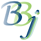# String Arrays

PRO/5 supports string arrays in the same manner as numeric arrays. Each element of a string array behaves just like any simple string variable. Unlike numeric arrays, when referencing a string array element, brackets must be used to enclose the subscripts. This is necessary to avoid ambiguity with substring specifications that must use parentheses.

A string array and a simple string variable with the same name are distinct.

## String Functions

There are many built-in functions in PRO/5 that return a string value. These functions may be used anywhere a string value may be used.

## String Expressions

PRO/5 supports the "+" operator, which performs string concatenation as follows:

B\$="ABC"
C\$="123"
LET A\$=B\$+"XYZ"+C\$

The resulting A\$ equals:

"ABCXYZ123"

## String Comparison

The relational operators described under Numeric Functions and Expressions may be used to compare two strings. PRO/5 compares the two strings, byte for byte, until two corresponding bytes are not equal or until it reaches the end of the shorter string. If the strings are identical to the shorter length, the shorter string is considered less than the longer string.

## Assigning Data to String Variables and Substrings

Data may be assigned to a string variable by means of an expression (i.e., the LET verb) or by means of an input verb. In any case there will be some string value as a source and some string variable as a destination. If the destination string variable has no substring specification then the entire variable is dynamically re-sized to match the length of the source string and the source string becomes the new value of the variable.

LET A\$="string value"

If the destination string variable has a substring specification, then the source string is either padded on the right with spaces, or truncated on the right to match the length of the destination substring. The length of the destination string variable is not changed.

LET A\$(4,6)="string value"
LET ARRAY\$(4,6)=C\$

In the first example above, only the value "string" is assigned since the destination, A\$(4,6), is only accepting 6 characters. Some programmers have mistakenly believed that PRO/5 would replace substring (4,6) with "string value" by shuffling the rest of A\$; therefore, changing the length of A\$. If this behavior is desired, it is necessary to build a new string.## BBj-Specific Information

In BBj 15 and higher, setting the OPENBASIC_ARRAY47 !COMPATsetting to TRUE enables an OpenBASIC compatibility setting to not report !ERROR=47 for substring out of bounds errors on empty array elements (i.e. array elements equal to ""). For example:

0010 print stbl("!COMPAT","OPENBASIC_ARRAY47=TRUE")

0020 dim a\$

0030 a\$="hello ",a\$="world"

0040 print a\$(2,3)+a\$+a\$

0050 print a\$(2,3)

0060 print stbl("!COMPAT","OPENBASIC_ARRAY47=FALSE")

0070 print a\$(2,3)+a\$+a\$

>run

OPENBASIC_ARRAY47=TRUE

hello world

OPENBASIC_ARRAY47=FALSE

!ERROR=47  (String index out of range: 1)

0070 print a\$(2,3)+a\$+a\$